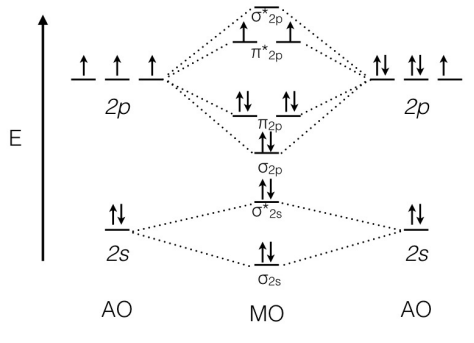# Problem: Utilize the molecular orbital diagram below to answer the following questions. How many electrons are present in antibonding molecular orbitals? (a) 2     (b) 4     (c) 6     (d) 8     (e) 10 What is the bond order for the molecule?(a) 3     (b) 2.5     (c) 2     (d) 1.5     (e) 1 What is the most likely formula for the molecule represented in the molecular orbital diagram?(a) O22−     (b) ONe     (c) ONe +     (d) OF     (e) OF +

###### FREE Expert Solution
92% (109 ratings)
###### Problem Details

Utilize the molecular orbital diagram below to answer the following questions.

How many electrons are present in antibonding molecular orbitals?

(a) 2     (b) 4     (c) 6     (d) 8     (e) 10

What is the bond order for the molecule?

(a) 3     (b) 2.5     (c) 2     (d) 1.5     (e) 1

What is the most likely formula for the molecule represented in the molecular orbital diagram?

(a) O22−     (b) ONe     (c) ONe +     (d) OF     (e) OF +What scientific concept do you need to know in order to solve this problem?

Our tutors have indicated that to solve this problem you will need to apply the MO Theory: Heteronuclear Diatomic Molecules concept. You can view video lessons to learn MO Theory: Heteronuclear Diatomic Molecules. Or if you need more MO Theory: Heteronuclear Diatomic Molecules practice, you can also practice MO Theory: Heteronuclear Diatomic Molecules practice problems.

What is the difficulty of this problem?

Our tutors rated the difficulty ofUtilize the molecular orbital diagram below to answer the fo...as high difficulty.

How long does this problem take to solve?

Our expert Chemistry tutor, Jules took 2 minutes and 44 seconds to solve this problem. You can follow their steps in the video explanation above.

What professor is this problem relevant for?

Based on our data, we think this problem is relevant for Professor Ray's class at UCSD.